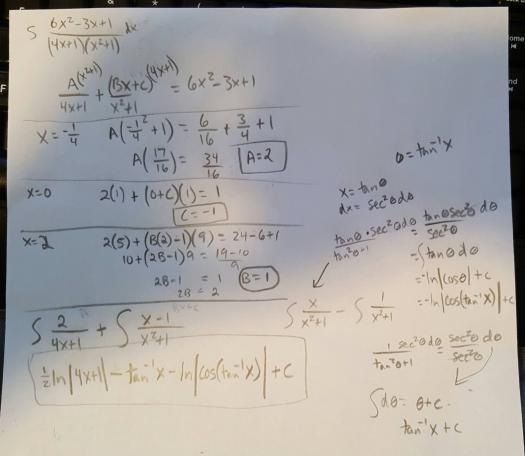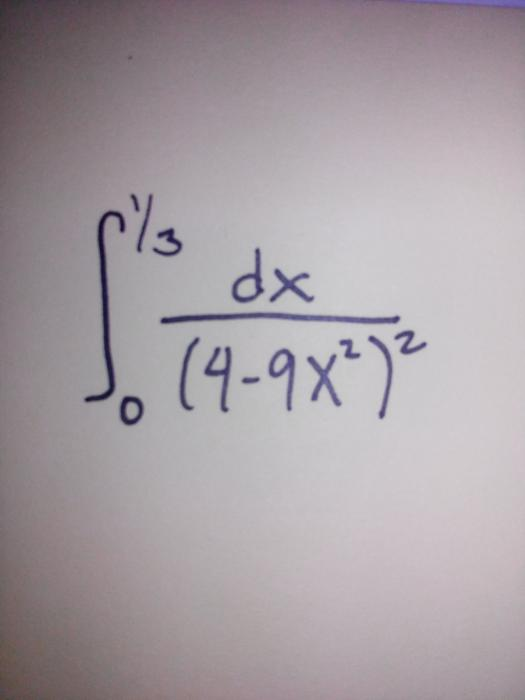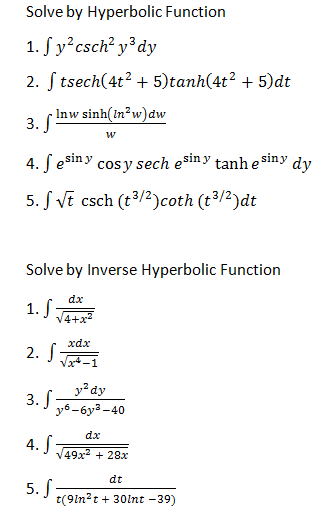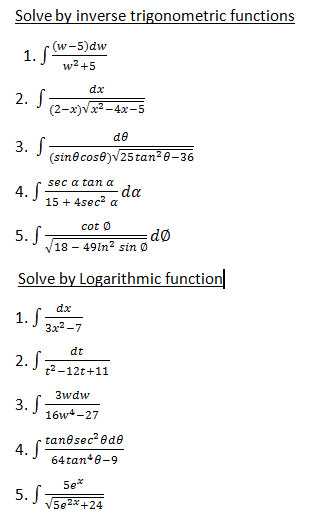# Integration

## Differential Equation: $y' = x^3 - 2xy$, where $y(1)=1$ and $y' = 2(2x-y)$ that passes through (0,1)

Can anyone solve this D. E.?

y' = x^3 - 2xy, where y(1)=1

and

y' = 2(2x-y) that passes through (0,1)## Integral Calculus: $\displaystyle \int \csc^3 x \, \cos^3 x dx$

Integral of csc^3 cos^3 xdx## Integration by Substitution

Kinda need some help.## Integral Calculus: $\displaystyle \int \ln \left[ x+(1-x)^{1/2} \right] dx$

Pa help po sana dito sa question na to.

(integral of) ln (x+(1-x)^(1/2))dx

## check my solution: $\displaystyle \int \dfrac{(6x^2 - 3x + 1) dx}{(4x + 1)(x^2 + 1)}$

hey...can anyone here check my solution to the problem??not sure whether I got it correctly and do not want to pay https://www.chegg.com/ or http://yourhomeworkhelp.org for the thing I practically did myselfthanks

## trigonometric function: $\displaystyle \int \dfrac{dx}{\cos(x) \sin(x)}$

∫dx/cos(x)sin(x)## definite integral hirap po talaga hindi ko masagot## patulong po Hyperbolic and Inverse Hyperbolic functions problems (Integral Calculus)## patulong po i solve tong mga problems (Integral Calculus)## ask ko lang po kung panong gagawin ko sa equation na to: integration of

(3-x)dx/ (x^2-6x-9)^5/2

## Pages